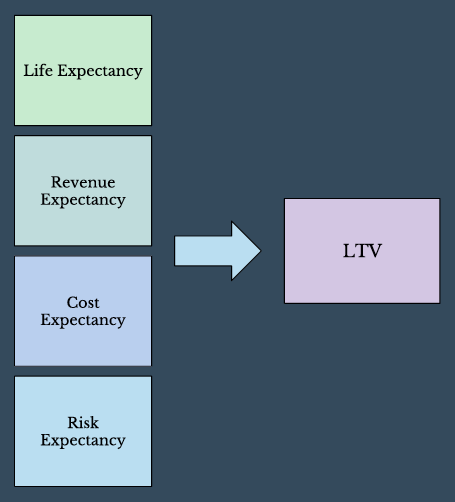# The Best LTV Formula for Subscription Businesses

Written by:

In our guide How To Measure LTV, I explained the four elements that go into LTV to give you a clear conceptual understanding of LTV.Now, let’s take a look at the actual mathematical formula that Zuora recommends you use to calculate LTV. Fortunately, for companies running subscription business, the formula only involves simple arithmetic.

The formula is:

Customer Lifetime Value (\$) = Current Recurring Revenue (\$) x Gross Profit Margin x Account Retention Rate / (1 + Discount Rate – Net MRR Retention)

Let’s imagine you operate a B2C monthly subscription business with the following metrics:
Monthly Recurring Revenue = \$9.99
• Gross Profit Margin = 80%
• Monthly Customer Account Retention Rate = 75%
• Discount Rate = 5%
• Net MRR Retention Rate = 85%

LTV would be calculated as:
Customer Lifetime Value (\$) = \$9.99 x 0.8 x 0.75 / (1 + 0.05 – 0.85) = \$5.99 / 0.2 = \$29.97

Over the course of four months (the life expectancy calculated using 1 / Churn Rate or 1/0.25), you can expect \$29.97 in profit from the customer.

### Other Formulas

There are a handful of alternate formulas that analysts use to calculate LTV. One of the most common alternative formulas is: LTV = \$ MRR / Churn Rate

This formula lacks two of the four critical elements: Cost Expectancy and Risk Expectancy. You should know by looking at this formula to use it at your own caution. While it may offer value in doing a relative future revenue comparison across your accounts, it would not have value as the basis of spending decisions because it aggressively overestimates the actual future value of one of your customers.

Another concept that I hear about the LTV metric from some companies is that they calculate it as a customer’s net payments lifetime-to-date or the sum of a customer’s net payments lifetime-to-date plus the LTV metrics described in this paper.

These are all interesting and valid ways to look at and segment your customers — especially from the perspective of developing customer loyalty programs based on rewarding customers for a steady financial relationship with you. That said, such a lens would offer misguided help on estimating the future value of your customers.